Function Repository Resource:

# ProjectionMatrix

Compute the projection matrix for a given vector space

Contributed by: Rauan Kaldybayev
 ResourceFunction["ProjectionMatrix"][A] returns the projection matrix onto the column space (range) of an m×n matrix A, with m≥n. ResourceFunction["ProjectionMatrix"][A,W] returns the projection matrix onto the column space (range) of A with a specified metric W.

## Details

The metric W should be a positive-definite matrix.
ResourceFunction["ProjectionMatrix"][A,W] returns the projection matrix for the case when the inner product of two vectors x and y equals xT.W.y for some positive-definite matrix W.

## Examples

### Basic Examples (3)

Compute the projection matrix onto the column space of a matrix:

 In:=Out=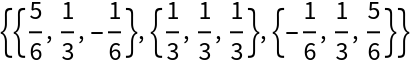Project a vector onto this vector space:

 In:=Out=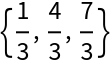Visualize:

 In:=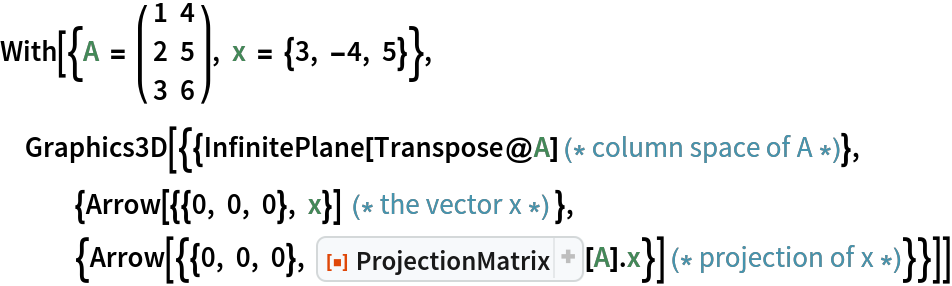Out=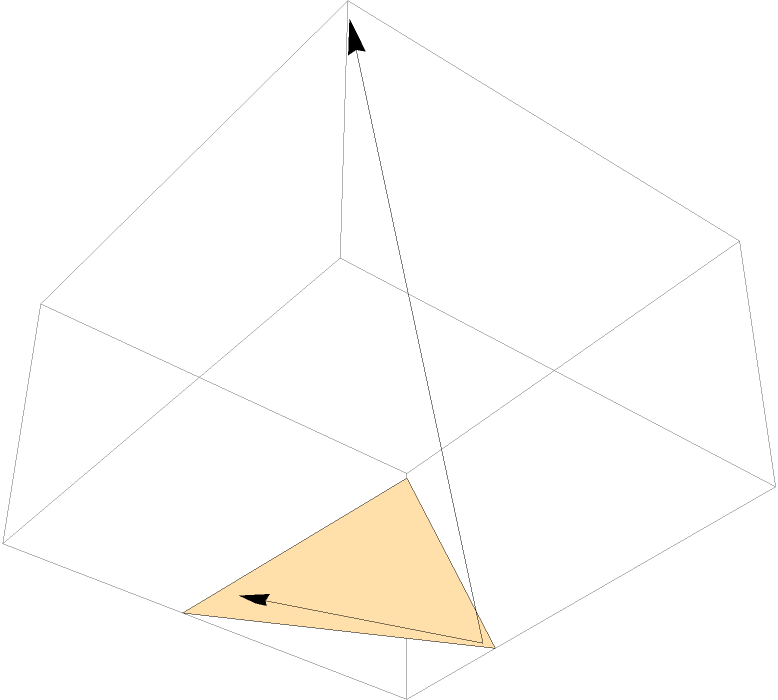### Scope (3)

Compute the projection matrix for the case when the dot product between a pair of vectors x and y is given by xT.W.y:

 In:=Out=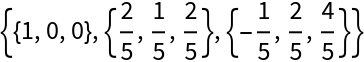Compute the projection matrix onto the column space of a singular square matrix:

 In:=Out=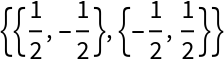Compute the projection matrix onto the column space of a symbolic real-valued matrix:

 In:=Out=### Properties and Relations (2)

ProjectionMatrix[Transpose@{v}].u is equivalent to Projection[u,v]:

 In:=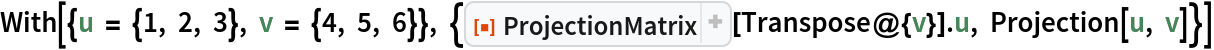Out=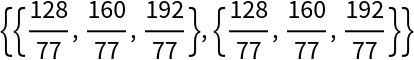ProjectionMatrix[Transpose@{v},W].u is equivalent to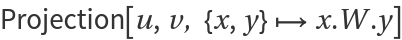:

 In:=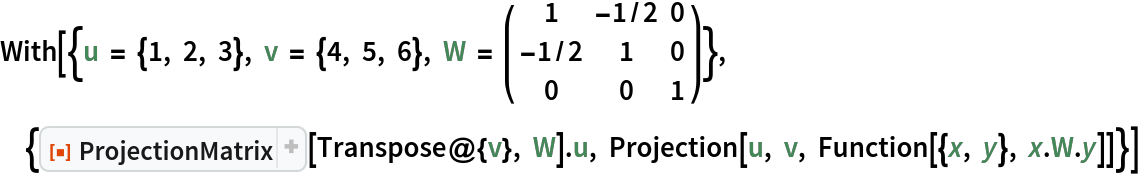Out=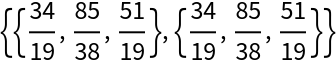### Possible Issues (1)

ProjectionMatrix can fail with badly conditioned numerical matrices. Consider Läuchli’s example. The output of the function may differ depending on whether the input is given in exact numbers or in MachinePrecision numbers:

 In:=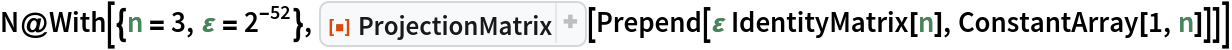Out=In:=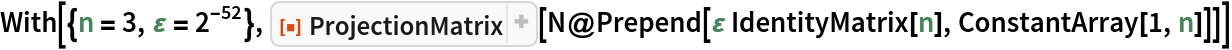Out=## Publisher

Wolfram Summer Camp

## Version History

• 1.0.0 – 19 January 2022